Next: Depolarizing Effects Up: Introduction Previous: Polarization of Electron Beams   Contents

## Spin Dynamics

The electron spin interacts with the electromagnetic field through the magnetic moment associated with the spin: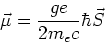(6)

where g is the gyromagnetic ratio of the electron . The deviation of this value from 2 is the anomalous magnetic moment of the electron: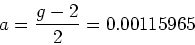(7)

We consider an electron at rest in a magnetic field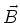; the precession equation of motion for the spin is the Larmor equation: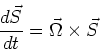(8)

with the angular velocity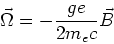(9)

Using Lorentz transformations the last equations can be rewritten  for a relativistic electron moving in the electromagnetic field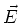andin an accelerator: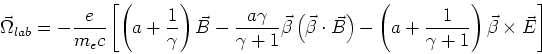(10)

which, when substituted into equation 8 (where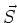is in the rest frame of the electron) is called the Thomas-BMT Equation where BMT stands for Bargman, Michel, Telegdi .

In a storage ring like SLS several types of fields are applied to the electron and are seen by the circulating particle periodically with period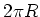, therefore it is more convenient to change the time variable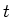into the traveled distance of the electron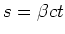. Most of the applied fields (quadrupole or sextupole magnets) only have an effect on an electron's orbit if its trajectory deviates from the designed circular orbit. The ideal electron traveling along the designed orbit will only see the guiding magnetic dipole field with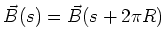as well as the accelerating electric field. The accelerating field however, does not cause spin precession on the ideal electron because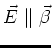. So we finally derive the spin precession of a highly relativistic (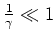) electron to be: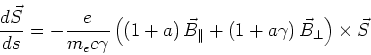(11)

where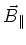and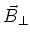are the magnetic fields parallel and perpendicular to the trajectory. In a flat machine (like SLS) the ideal electron sees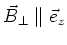(leading to Thomas precession) and vanishing(leading to Larmor precession)  . We now are able to write the spin precession frequency in the particle's rest frame (i.e. in machine coordinates):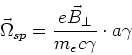(12)

with the relativistic cyclotron frequency (the revolution frequency of the particles in the storage ring)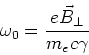(13)

and the spin tune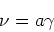(14)

describing the number of times the spin vector precesses around the bending field vector for one turn of the particle around the ring. This is shown schematically in Figure 1. The relationship between spin precession frequency and energy of the particles (equation 12) is the mechanism which is used to determine the precise energy of the electrons in the SLS storage ring (see chapter 3.1).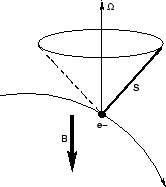Figure 1: Precession of the electron spin around the main bending magnet field.

A solution to equations 11 and 12 for the flat machine therefore leads to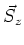(the component of the spin in direction of the main bending field) remaining constant. The other components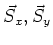are statistically distributed among the electrons in the beam. After integration over all the electrons in the ensemble the only remaining component is. The time-integration of the remaining component over the ensemble leads to a constant degree of polarization.Next: Depolarizing Effects Up: Introduction Previous: Polarization of Electron Beams   Contents
Simon Leemann
2002-03-15